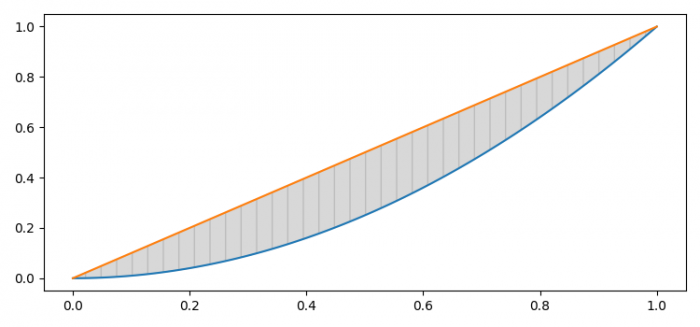# Find the area between two curves plotted in Matplotlib

To find the area between two curves plot in matplotlib, we can take the following steps

• Set the figure size and adjust the padding between and around the subplots.
• Create x, c1 and c2 data points using numpy.
• Plot (x, c1) and (x, c2) using plot() methods.
• Fill the area between the two curves, c1 and c2, with grey color and hatch "|", using fill_between() method.
• To display the figure, use show() method.

## Example

import matplotlib.pyplot as plt
import numpy as np
plt.rcParams["figure.figsize"] = [7.50, 3.50]
plt.rcParams["figure.autolayout"] = True
x = np.linspace(0, 1, 100)
c1 = x ** 2
c2 = x
plt.plot(x, c1)
plt.plot(x, c2)
plt.fill_between(x, c1, c2, color="grey", alpha=0.3, hatch='|')
plt.show()

## Output